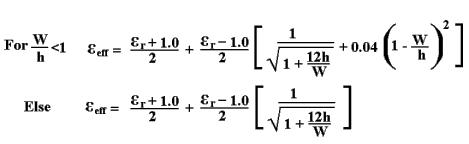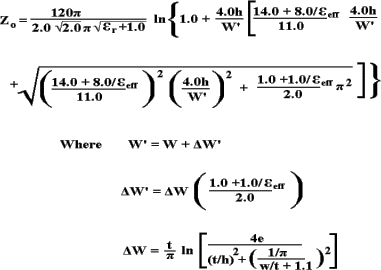# Microstrip Transmission Line Characteristic Impedance Calculator Using an Equation by Brian C Wadell

The characteristic impedance (Zo) of a microstrip transmission line can be calculated using the active calculator or the equations at the bottom of the page.INPUT DATA Relative Dielectric Constant ( εr ): Track Width: mm Track Thickness: mm Dielectric Thickness: mm
 RESULTS Effective Dielectric Constant (εeff): Characteristic Impedance (Zo): Ohms

This calculator uses JavaScript and will function in most modern browsers. For more information see About our calculators

The following equations were derived from equations used in an article by Rick Hartley http://www.jlab.org/accel/eecad/pdf/050rfdesign.pdf It is not too clear from the article by Rick Hartley where to use εr and where to use εeff (although there is a note to use eeff instead of er). The substitutions selected give results that are almost identical to the "benchmarks" in http://www.polarinstruments.com/support/cits/IPC1999.pdf and these benchmarks were obtained by numerical methods. The accuracy of results obtained from this calculator can be checked by making a sample PCB and measuring the resulting impedance. Guidance for this can be found at Measuring Characteristic Impedance of PCB tracks using a Vector Network AnalyserWhere εr is the Relative Dielectric Constant

W is the Width of track

t is the Thickness of track (1 oz/ft2 = 0.035 mm)

h is the Thickness of dielectric

The above equations are also in Transmission Line Design Handbook by Brian C. Wadell, Artech House 1991, with some differences in calculating εeff. The main equation is attributable to Harold A. Wheeler and was published in, "Transmission-line properties of a strip on a dielectric sheet on a plane", IEEE Tran. Microwave Theory Tech., vol. MTT-25, pp. 631-647, Aug. 1977. The effective dielectric constant equation is from: M. V. Schneider, "Microstrip lines for microwave integrated circuits," Bell Syst Tech. J., vol. 48, pp. 1422-1444, 1969.

This calculator is provided free by Chemandy Electronics in order to promote the FLEXI-BOX

Return to Calculator Index